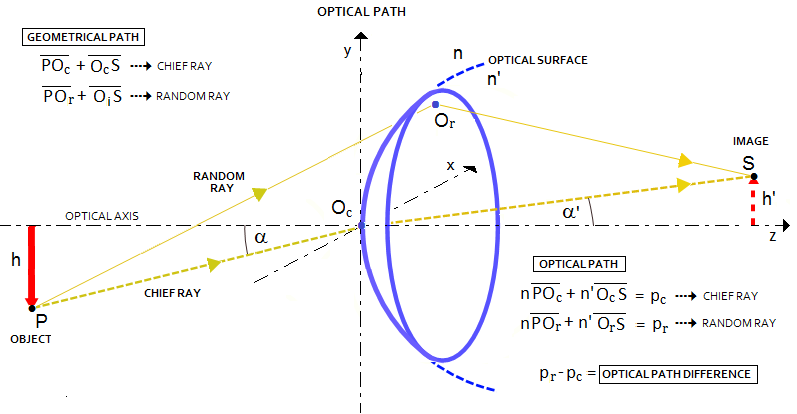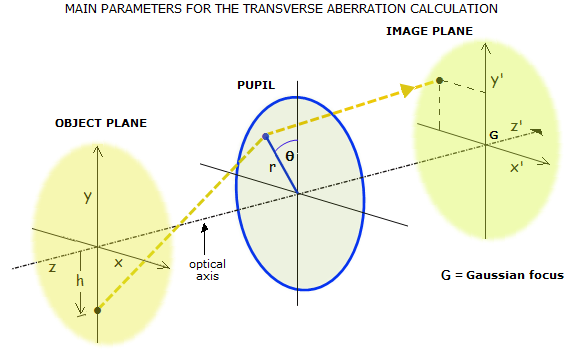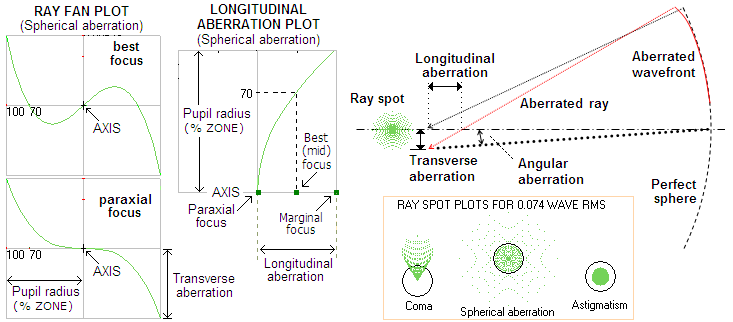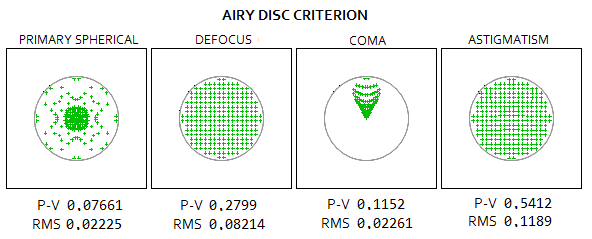telescopeѲptics.net          ▪▪▪▪                                             CONTENTS

# 3.2. Ray (geometric) aberrations

Wavefront deformations are inevitably accompanied by disturbance of rays - by definition straight lines, or light paths - orthogonal to the wavefront surface. Disturbance of rays caused by wavefront errors manifests as angular deviation of the aberrated ray, producing linear ray deviation in the image plane, usually either from Gaussian image point, or from best (diffraction) focus.

Note that the condition for light rays to remain straight is homogenous media. This is the consequence of Fermat's principle, which in its elementary form states that light ray follows a path that requires the least amount of time to connect two separate points. For instance, a ray that connects point-source with its conjugate point (focus) formed by a positive lens will follow different paths, depending on its initial orientation. A ray arriving at the front lens surface along the path coinciding with its optical axis, will proceed straight toward the focus. On the other hand, a ray arriving at the front lens surface at an angle, will bend (refract) entering the lens, according to the law of refraction, reach the opposite lens surface along the straight line, refract again when exiting the lens, and arrive at its intersection with the chief ray (i.e. Gaussian image point) following a straight line.

Whether the point of intersection of all the rays will coincide with the lens focus, is determined by the optical properties of the lens. In order for that to happen, optical path length for all rays needs to remain constant. Note that optical path length differs from path's geometric length, being subject to the media refractive index: optical path length through a media of refractive index 2 is, for instance, twice its geometric length. This is why rays having different geometric path lengths traveling through a lens objective, still can have identical optical path lengths, enabling wave points in phase to arrive at the focal point simultaneously.Variations in optical path length of individual rays, on the other hand, result in optical path difference for wave points in phase, wavefront deformation, and wave phase mismatch at the focal point. While optical path length and optical path difference resulting from it are obtained from the geometry of rays in a given optical system, the focal zone ray geometry alone is not directly related to these categories, hence it is not a reliable predictor of the level of optical quality. Ray aberrations are merely linear ray deviations from the focus point, thus not conveying any information on the actual energy distribution around it.

Yet, ray geometry offers convenient way for determining basic properties of an optical system. Gaussian, or "paraxial" approximation, introduced by Carl Friedrich Gauss, follows rays close enough to the axis that sines and tangents can be replaced by the angles themselves; this is so called "first-order" optics, used to determine paraxial focus location. Gaussian focus coincides with the focus of a perfect optical surface, or system. On the other hand, best or diffraction focus is the location of best actual image, which is in the presence of aberrations often displaced from Gaussian focus.Linear ray deviation from the focus point has two forms: longitudinal, measured along the chief (central) ray, from where it intersects with aberrated ray to the focus location, and transverse, measured from the focal point to the ray height above or below it in the focal plane. The former defines longitudinal ray aberration, and the latter transverse ray aberration (FIG. 22, center).

Transverse ray aberrations can be presented graphically by plotting points of intersection of a selected number of rays with the focal plane. These are referred to as ray spot or ray spot plot (FIG. 22, center) also, ray spot diagram, or geometric blur. Another form of presenting transverse ray aberration is ray fan plot (FIG. 22, left); similarly, longitudinal aberration can be plotted as a function of the ray height in the pupil (FIG. 22, right). Transverse ray deviations can be expressed in angular form, given by the size of transverse aberration relative to the system focal length.FIGURE 22
: Main forms of ray (geometric) aberration and its presentation. RIGHT, aberrated wavefront deviates from its perfect reference sphere by being more strongly curved toward the edge. As a result, marginal rays focus shorter than central rays, with the distance from the aberrated to proper focus constituting longitudinal aberration, and the radial height of the aberrated ray in the image plane constituting transverse aberration (in this illustration, both are negative, the former being oriented to the left, and the latter having the radius on the opposite side of the pupil radius of origin). Ray spot plot presents ray distribution in the image plane; in the square, ray spot plots for the conventional "diffraction limited" level (o.074 wave RMS, 0.80 Strehl) of coma, spherical aberration and astigmatism (0.42, 0.25 and 0.37 wave P-V, respectively) vs. Airy disc (black circle). The spot size changes in proportion to the P-V/RMS wavefront error. LEFT, ray fan plots representing transverse aberration as a function of the ray height in the pupil. The top graph is a ray fan plot for transverse primary spherical aberration at the best focus location (at the illustration in the center, that would be mid-point of the longitudinal aberration). The plot shows that rays from below 70% zone focus before best focus location, thus intersecting the image plane at the opposite side of the axis; the 70.7% zone ray focus coincides with the best focus location (thus the transverse aberration is zero), while rays from the higher zones focus longer, intersecting the image plane at the same side of the axis where is their pupil radius of origin. Bottom plot is a ray fan plot for paraxial focus location, for which all rays other than paraxial focus shorter, the higher zone, the more so (paraxial blur is larger than best focus blur by a factor of 2, which would show on the vertical numerical scale). CENTER, longitudinal aberration plot (also for primary spherical aberration) shows focus location as a function of zonal height in the pupil; since longitudinal aberration here changes with the square of zonal height, 70% zone ray focuses at just short of the mid point of the longitudinal aberration span.

As already mentioned, ray aberrations are not reliable indicator of energy re-distribution within diffraction pattern, which is the key information needed to access the effect of aberration on image quality. There is simply no direct relation between either geometric or angular form of ray aberrations with the physical aspect of imaging - that is, wave interference. However, they can give a rough impression of imaging quality and, when resulting from known forms of wavefront aberrations, their relation to the RMS wavefront error is also known and, thus, can be associated with a specific degree of image deterioration.As an initial indicator of optical quality, optical designers often consider a system close to "diffraction-limited" if its ray spot radius doesn't exceed the Airy disc radius. Called the Golden rule of optical design, it is still a very loose indicator of optical quality which, depending on the type of aberration, can be associated with aberration levels of anywhere from ~0.5 to 0.999 Strehl. Ray spot diagrams at left are good illustration of how unreliable is geometric blur size as a criterion of optical quality. If coma spot would have been made large as Airy disc, it would still be significantly lower aberration level than the "diffraction limited" 0.80 Strehl. At that level, coma blur is 1.56 times, primary spherical 3.4 times, astigmatism 0.6 and defocus 0.8 times the Airy disc diameter.

A more recent form of the geometric blur presentation, the RMS spot size, gives statistical spot size expressing average distance of a large number of individual rays from the central spot. It is not to be confused with the RMS wavefront error, a much more accurate indicator of optical quality. While the RMS spot size is probably somewhat less uncertain as an optical quality indicator than a plain geometric blur size, it is still mainly determined by the geometric blur size, hence not significantly more reliable. For instance, geometric blur size for paraxial, marginal and best focus for spherical aberrations relate as 1:0.385:0.5, respectively; their RMS spot sizes relate (in the same relative units) as 0.5:0.289:0.204, while the respective RMS wavefront errors relate as 1:1:0.25.

Directly related to the RMS spot size is geometric PSF (Point Spread Function), determined by the distribution of rays in the image plane, so called ray or geometric irradiance (as opposed to actual energy distribution given by diffraction PSF). The point around which the rays are balanced quantitatively is called centroid (center of gravity). For radially symmetrical aberrations like spherical, or those with a central symmetry, like astigmatism, it coincides with the position of the chief (central) ray; for asymmetric aberrations, such as coma, it is shifted from it according to the form of ray distribution.